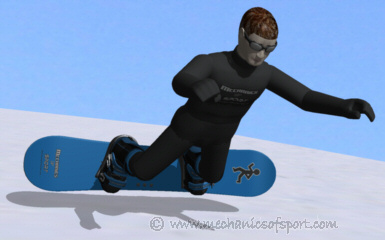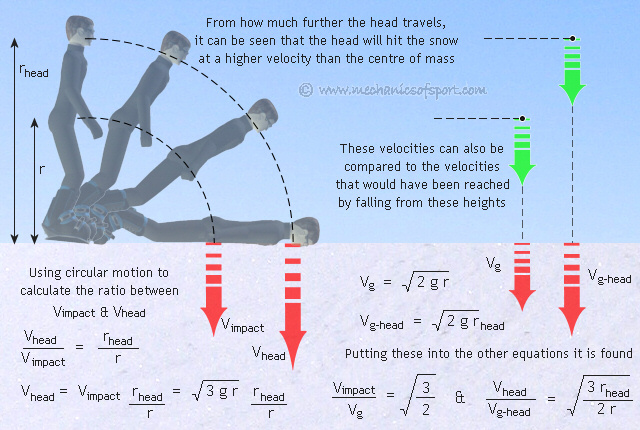SNOWBOARDING

# Mechanics of Catching an EdgeThis page shows how the velocities involved in catching an edge can be estimated. By looking at the mechanics of a pivot edge catch, we see that the velocities can be reasonably predicted. The calculations also show how we can affect an edge catch, as well as work out the transition velocity between a pivot and flying edge catch.

To calculate the velocities a few assumptions have to be made to base the models and calculations on. The main assumptions used are that all forces will act on the centre of mass of the rider, that all energy is conserved throughout an edge catch, and in most cases that the edge catch happens on a flat part of a slope.

## The Pivot Edge Catch

The pivot edge catch is where the body is thrown at the snow with the edge acting as a pivot. There is a limit to the initial velocity for a pivot edge catch though, as if a snowboarder is going too fast, their rotation will take the edge of the snowboard out of the snow, stopping the pivot effect, and turning the motion into a flying edge catch.

### Maximum Velocity for a Pivot Edge Catch

From the diagram below you can see that if the centrifugal force created is greater than gravity the edge will release upwards almost instantly and the snowboarder will come into the air. The maximum sideways velocity at which the edge stays in the snow (Vmax) can be worked out. Then Vmax can be used to find out the worst case scenario for this type of edge catch.### Impact Velocity

Now the maximum entrance velocity for a pivot edge catch is known, lets work out the impact velocities that it creates. To do this the assumption that all energy is conserved during the crash is made, which means that the initial kinetic and potential energy, will both be kinetic energy at impact. This assumption also means that there is no wind resistance, that the edge will stay in the snow all the way until impact (in real life the toes or heels will push it out of the snow a few degrees earlier), and that the centre of mass will go all the way to level with the snow (again not quite true). From this the impact velocity of the centre of mass (Vimpact) can be calculated.The snowboarder's head has to travel further to hit the snow than their centre of mass does, yet they still hit the snow at the same time. This means that the head will be going faster than the centre of mass when it hits the snow. By using using the distances from the snow to the centre of mass and the head, how much faster the head will be traveling can be calculated.### General Maximum Velocities for a Pivot Edge Catch on a Flat Slope

By putting the force of gravity and the measurements of an average person into these equations, the general resultant velocities can be calculated, and comparisons can be made.From this we find that the sideways velocity at which the edge throws you to the ground the hardest is roughly 11km/h (7mph), and that your head will hit the snow at 32km/h (20mph). This means your head will hit the snow 1.6 times harder than if you had fallen from your head height. Overall these figures show that you do not have to be going very fast to really slam, and that although you would be unlucky for these impact velocities to seriously injure you, they will hurt. If you catch an edge at this speed it will also only be about a third of a second until you to hit the snow, which when you consider the average poised reaction time is between a fifth and a quarter of a second, it means that if you're not expecting anything you can easily hit the snow before you even realise you had caught an edge.

### What Differences Can You Make?

From looking at the equations, we can see how we are able to effect the outcome from an edge catch. Firstly if your centre of mass is lower when you catch an edge (i.e. if you've crouched down), you will not hit the ground as hard, as there is less gravitational potential energy. Lowering your centre of mass also makes Vmax decrease, which makes it more likely that and edge catch would be a flying edge catch not a pivot edge catch. Another thing the equations show is that after you have caught an edge, if you lift your arms over you head before you hit the snow, this will move your centre of mass further along you body and your head will not hit quite as hard.

## The Flying Edge Catch

A flying edge catch has more energy to it than a pivot edge catch, assuming only velocity has changed, but can be less painful as the energy is transferred differently. The mechanics of a flying edge catch are more complicated than a pivot edge catch, and there are even variables that make different things able to happen at the same speed. As simple equations and basic assumptions cannot be used to cover the variables and accurately calculate what will happen, it is better just to show the characteristics of a flying edge catch without trying to calculate much.